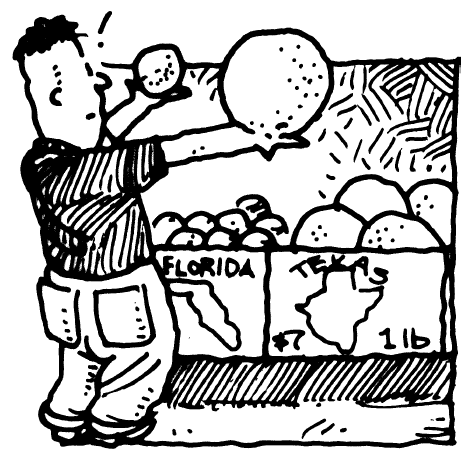### Home > PC > Chapter 5 > Lesson 5.1.1 > Problem5-8

5-8.

If $\int _ { - 1 } ^ { 3 } f ( x ) d x = 17$ (or $A\left(f, − 1 ≤, x ≤ 3\right) = 17$) and $h\left(x\right) = f\left(x\right) + 5$,

What does equal $\int _ { - 1 } ^ { 3 } h ( x ) d x$?

The new function is the same as the old function raised up $5$ units. What is the width of the region?
How much more area does $h\left(x\right)$ have?